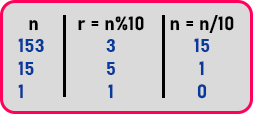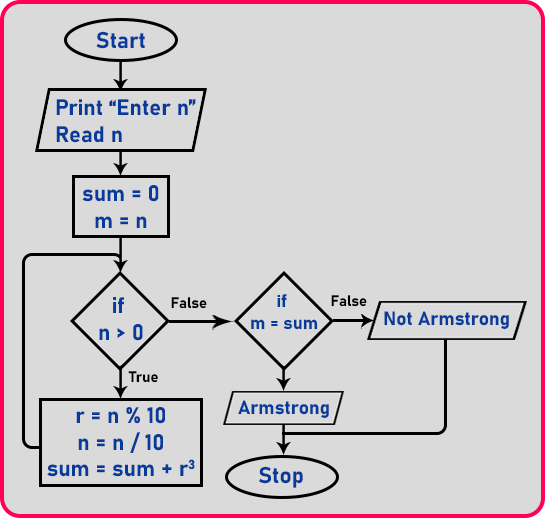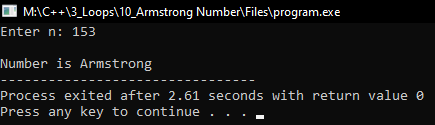# Armstrong Number using Loop in C++

## Armstrong Number using Loop in C++

In this article, I am going to discuss Armstrong Number using Loop in C++ with Examples. Please read our previous articles, where we discussed How to Display digits of a number using Loop in C++ with Examples. Here, we will write a program for checking if a number is Armstrong.

##### Armstrong Number:

Let first understand what is an Armstrong number. Suppose we have a number ‘n = 153’, now separate its digits as, ‘1’, ‘5’, and ‘3’. Now if we cube to all of the digits as,

13 = 1

53 = 125

33 = 27

And add these all ‘1 + 125 + 27 = 153’, after addition we get the number itself. So, the definition of Armstrong number is when the sum of the cubes of the digits of any number is equal to that number itself then it is known as Armstrong number.

Let’s see the procedure to check whether a given number is Armstrong or not. First of all, we need the digits of that number. As in the previous article we have already seen how to take out digits from a number. If you don’t know about it, check that article. So, for getting the last digit we used mod operator with ’10’, like the same number we are taking here,

153 % 10 = 3

Here ‘3’ is extracted from the number. Now we need previous digits also and we can get only those digits which are present at last in the number. We make ‘5’ as the last digit by using division with ‘10’ as

153 / 10 = 15

Here we got ‘15’ and the last digit is ‘5’. In this way, we take out all the digits and then add the cubes of these digits as

= 13 + 53 + 33

= 1 + 125 + 27

= 153

Below is the table of the above steps,We have seen the procedure, now let us look at the flowchart of Armstrong number.

##### Armstrong Number Flowchart:First, we will take the number as input from the user. Next, we take two variables ‘sum’ which will be initialized to ‘0’ and ‘m’ which will equal to the number. Next, we will check the condition ‘if (n > 0)’, if it will true then process below 3 steps:

1. r = n % 10 (To get the last digit of the number).
2. n = n / 10 (To make the previous digit as the last digit of the number).
3. Sum = sum + r3.

After these steps again return to the condition ‘if (n > 0)’, if it will false then check another condition ‘if (m = sum)’, if it will true then print ‘Armstrong’ or if it will false the print ‘Not Armstrong’. Now let us look at the program.

##### Program to check whether the number is Armstrong or not using loop in C++:
```#include <iostream>
using namespace std;
int main()
{
int n, r, sum = 0, m;
cout << "Enter n: ";
cin >> n;
cout << endl;
m = n;
while (n > 0)
{
r = n % 10;
n = n / 10;
sum = sum + r * r * r;
}
cout << "Number is ";
if (sum == m)
cout << "Armstrong";
else
cout << "not Armstrong";
return 0;
}
```
###### Output: